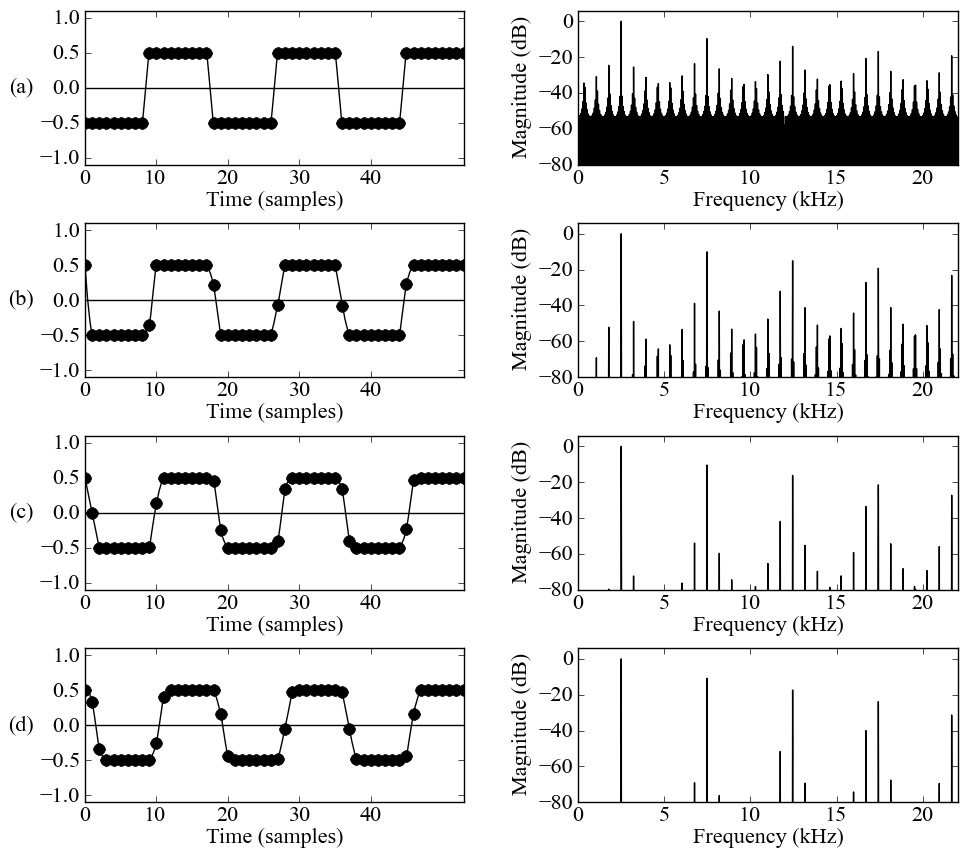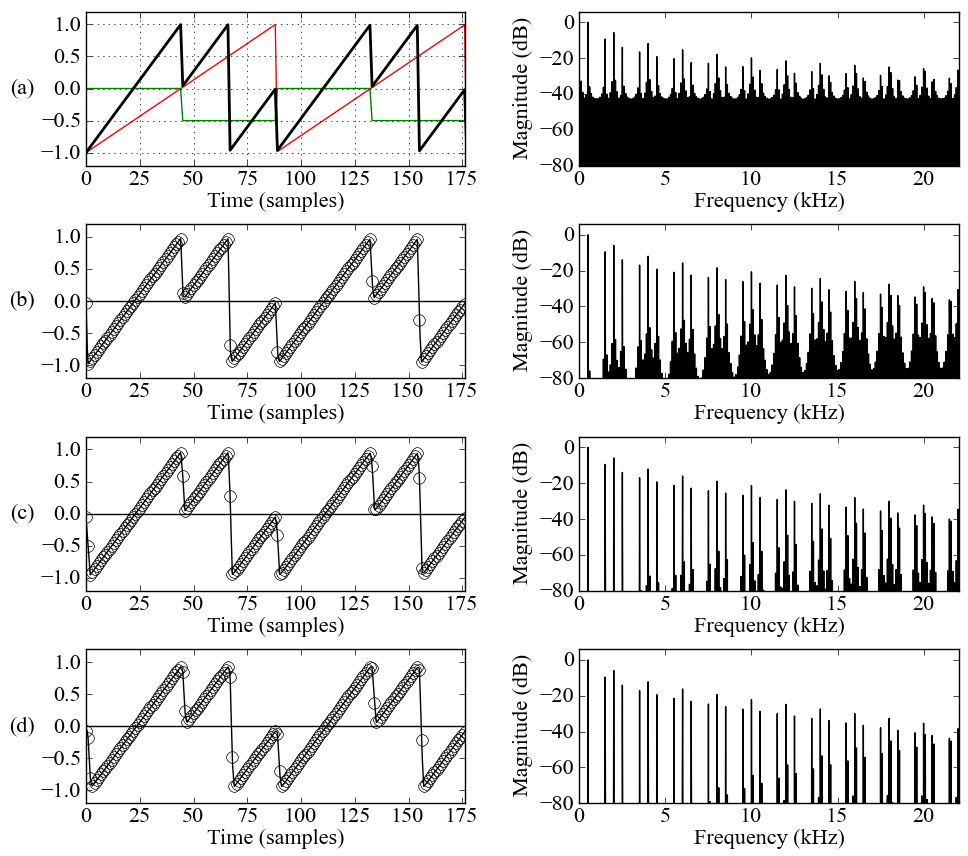# Polynomial Transition Regions (PTR)

“Reducing Aliasing from Synthetic Audio Signals using Polynomial Transition Regions,”
to appear in IEEE Signal Processing Letters.

Aalto University, School of Electrical Engineering, Dept. of Signal Processing and Acoustics, Espoo, Finland

#### Source Code

• Python source for the algorithms, figures and sound examples from the paper (includes additional examples discussed below).
• Benchmark code as Pure Data (Pd) externals

#### Audio Examples

The audio examples are 16-bit signed PCM wavs, sampling rate is 44.1 kHz.
Sound   Description   Parameters   Size (MB)
Square wave trivial (3s) - pause (1s) - PTR W = 3 (3s) f0 = 2490 Hz, r = 3.5 0.6
Pulse Width Modulation trivial (4s) - pause (1s) - PTR W = 3 (4s) f0 = 698 Hz, Triangular LFO = 0.25 Hz 0.78
XOR synthesis trivial (3s) - pause (1s) - PTR W = 3 (3s) f0 = 500 Hz, see below for other parameters 0.6
Transients Fig.3: DPW N = 3 (6s) - pause (1s) - PTR W = 2 (6s) f0 = 500 Hz to 750 Hz, ramp-like fm = 10 Hz 1.09
Hardsync Fig.4: trivial (3s) - pause (1s) - PTR W = 3 (3s) fmaster = 1660 Hz, r = 1.5 0.6
Hardsync sweep Fig.4: trivial (3s) - pause (1s) - PTR W = 3 (3s) fmaster = 1000 Hz → 1500 Hz, r = 3.5 0.6

#### DPW Correction Polynomials

DPW correction polynomials (Eq.7) as function of distance from the discontinuity D(n) = ϕ(n)P0 = ϕ(n)/T0.
 W Span Correction polynomial cW(D) 1 0…T0 –2D + 2 2 0…T0 D2 – 4D + 4 2 T0…2T0 –D2 + 2 3 0…T0 –D3/3 + 2 3 T0…2T0 2D3/3 – 3D2 + 3D + 1 3 2T0…3T0 –D3/3 + 3D2 – 9D + 9

#### Further Application Examples

##### Pulse Waveform

The figure below shows that the aliasing contained in the trivial square wave (a), f0 = 2490 Hz, fs = 44100 Hz is suppressed substantially when using PTR of (b) W = 1, (c) W = 2, and (d) W = 3. The transition polynomials can be derived from Table 1 in the paper, and they are available in the Python source code release. Sound examples of the pulse waveform depicted below and of a pulse-width modulated timbre are available in the Audio Examples section above.##### XOR Synthesis

XOR synthesis  applies bitwise exclusive-or operation between two audio signals. It is an efficient and versatile method, which unfortunately suffers from excessive aliasing. This has thus far reduced the usability of the method in musical audio contexts. PTR seems to mitigate the aliasing problems, opening the XOR method for further research efforts.

In the example below, bitwise XOR operation is applied between a bipolar sawtooth waveform (red, f0 = 500 Hz) and a square wave (green, f0 = 500 Hz), fs = 44100 Hz. The output waveform (thick black) onsists of two parts: a full-cycle ramp followed by its phase-shifted replica. As can be seen, although the output spectrum is contaminated with notorioius aliasing distortion, partials 2,6,10,14,... seems to be missing.This is indeed the case, as shown in the PTR cleanups (b) W = 1, (c) W = 2, and (d) W = 3. The process was two-pass: the algorithm computes first the trivial aliasing contaminated input signal (a), and then applies the scaled and offset transition polynomials to the transition regions, leaving the other parts of the original signal intact (as given by Eq.(8)).